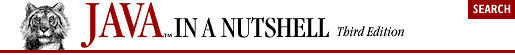home | O'Reilly's CD bookshelfs | FreeBSD | Linux | Cisco | Cisco Exam4.4. Dates and Times

Java uses several different classes for working with dates and times. The java.util.Date class represents an instant in time (precise down to the millisecond). This class is nothing more than a wrapper around a long value that holds the number of milliseconds since midnight GMT, January 1, 1970. Here are two ways to determine the current time:

long t0 = System.currentTimeMillis();      // Current time in milliseconds
java.util.Date now = new java.util.Date(); // Basically the same thing
long t1 = now.getTime();                   // Convert a Date to a long value

The Date class has a number of interesting-sounding methods, but almost all of them have been deprecated in favor of methods of the java.util.Calendar and java.text.DateFormat classes. To print a date or a time, use the DateFormat class, which automatically handles locale-specific conventions for date and time formatting. DateFormat even works correctly in locales that use a calendar other than the common era (Gregorian) calendar in use in much of the world:

import java.util.Date;
import java.text.*;

// Display today's date using a default format for the current locale
DateFormat defaultDate = DateFormat.getDateInstance();
System.out.println(defaultDate.format(new Date()));

// Display the current time using a short time format for the current locale
DateFormat shortTime = DateFormat.getTimeInstance(DateFormat.SHORT);
System.out.println(shortTime.format(new Date()));

// Display date and time using a long format for both
DateFormat longTimestamp =
DateFormat.getDateTimeInstance(DateFormat.FULL, DateFormat.FULL);
System.out.println(longTimestamp.format(new Date()));

// Use SimpleDateFormat to define your own formatting template
// See java.text.SimpleDateFormat for the template syntax
DateFormat myformat = new SimpleDateFormat("yyyy.MM.dd");
System.out.println(myformat.format(new Date()));
try {   // DateFormat can parse dates too
Date leapday = myformat.parse("2000.02.29");
}
catch (ParseException e) { /* Handle parsing exception */ }

The Date class and its millisecond representation allow only a very simple form of date arithmetic:

long now = System.currentTimeMillis();         // The current time
long anHourFromNow = now + (60 * 60 * 1000);   // Add 3,600,000 milliseconds

To perform more sophisticated date and time arithmetic and manipulate dates in ways humans (rather than computers) typically care about, use the java.util.Calendar class:

import java.util.*;

// Get a Calendar for current locale and time zone
Calendar cal = Calendar.getInstance();

// Figure out what day of the year today is
cal.setTime(new Date());                        // Set to the current time
int dayOfYear = cal.get(Calendar.DAY_OF_YEAR);  // What day of the year is it?

// What day of the week does the leap day in the year 2000 occur on?
cal.set(2000, Calendar.FEBRUARY, 29);           // Set year, month, day fields
int dayOfWeek = cal.get(Calendar.DAY_OF_WEEK);  // Query a different field

// What day of the month is the 3rd Thursday of May, 2001?
cal.set(Calendar.YEAR, 2001);                      // Set the year
cal.set(Calendar.MONTH, Calendar.MAY);             // Set the month
cal.set(Calendar.DAY_OF_WEEK, Calendar.THURSDAY);  // Set the day of week
cal.set(Calendar.DAY_OF_WEEK_IN_MONTH, 3);         // Set the week
int dayOfMonth = cal.get(Calendar.DAY_OF_MONTH);   // Query the day in month

// Get a Date object that represents 30 days from now
Date today = new Date();                   // Current date
cal.setTime(today);                        // Set it in the Calendar object